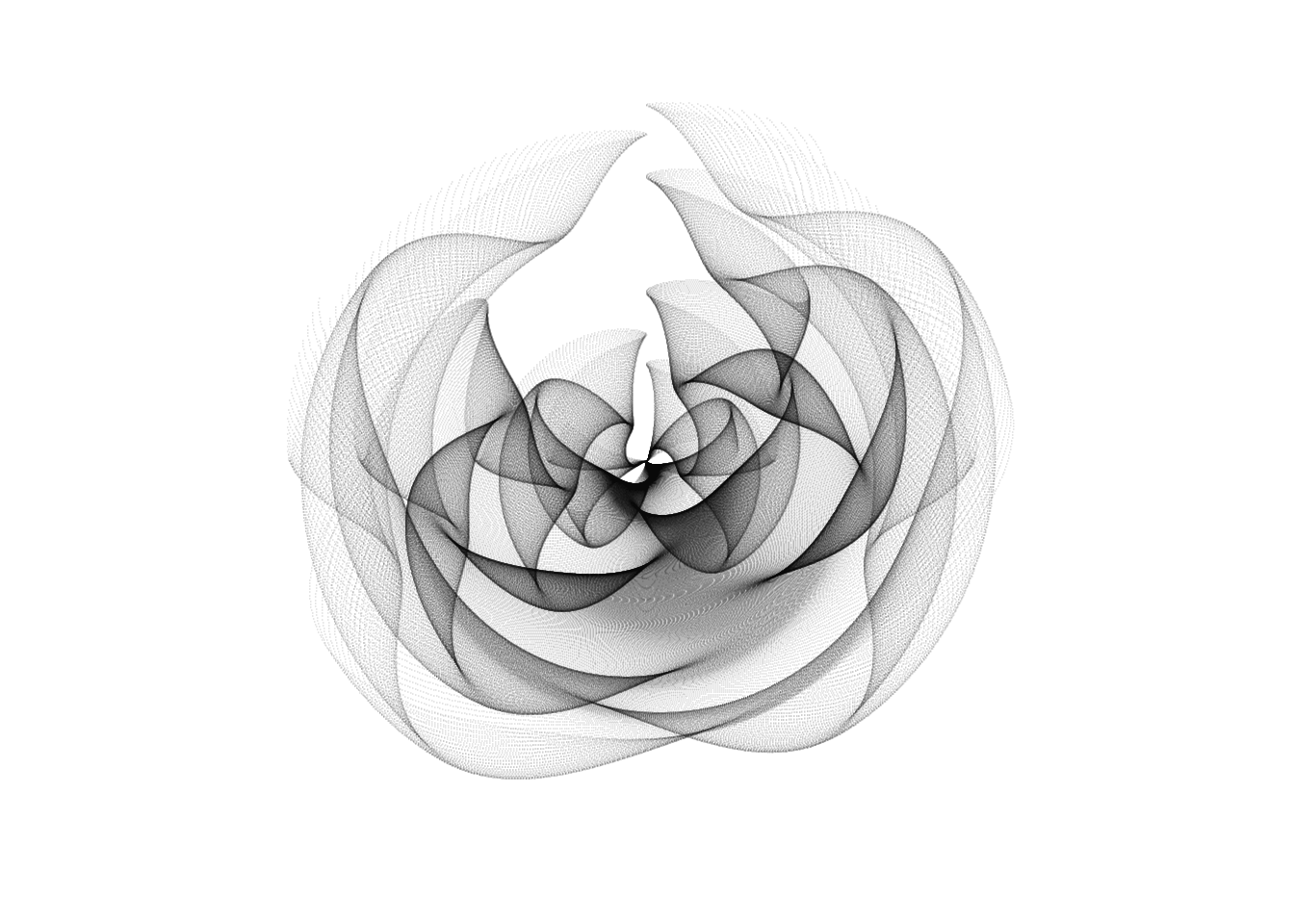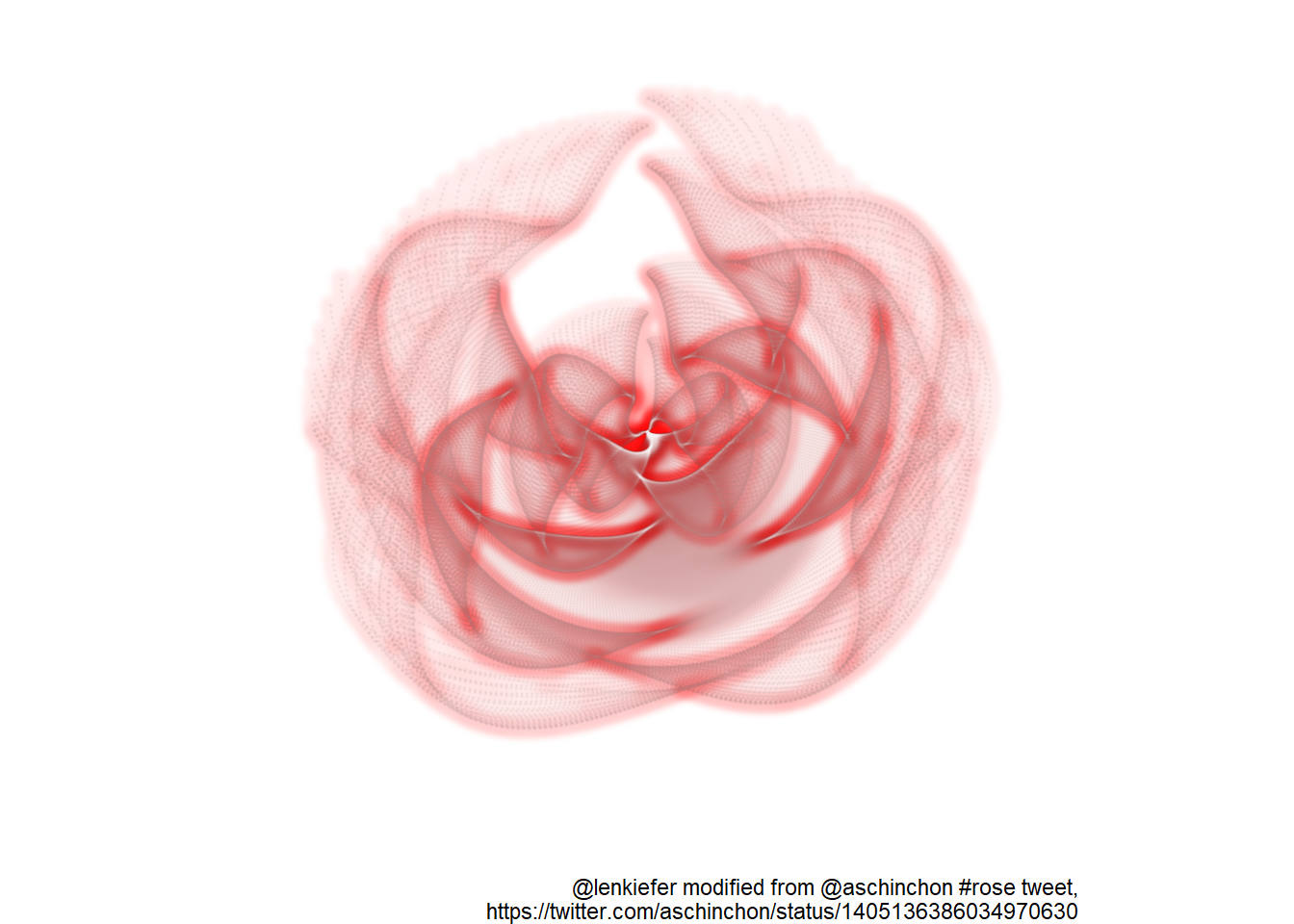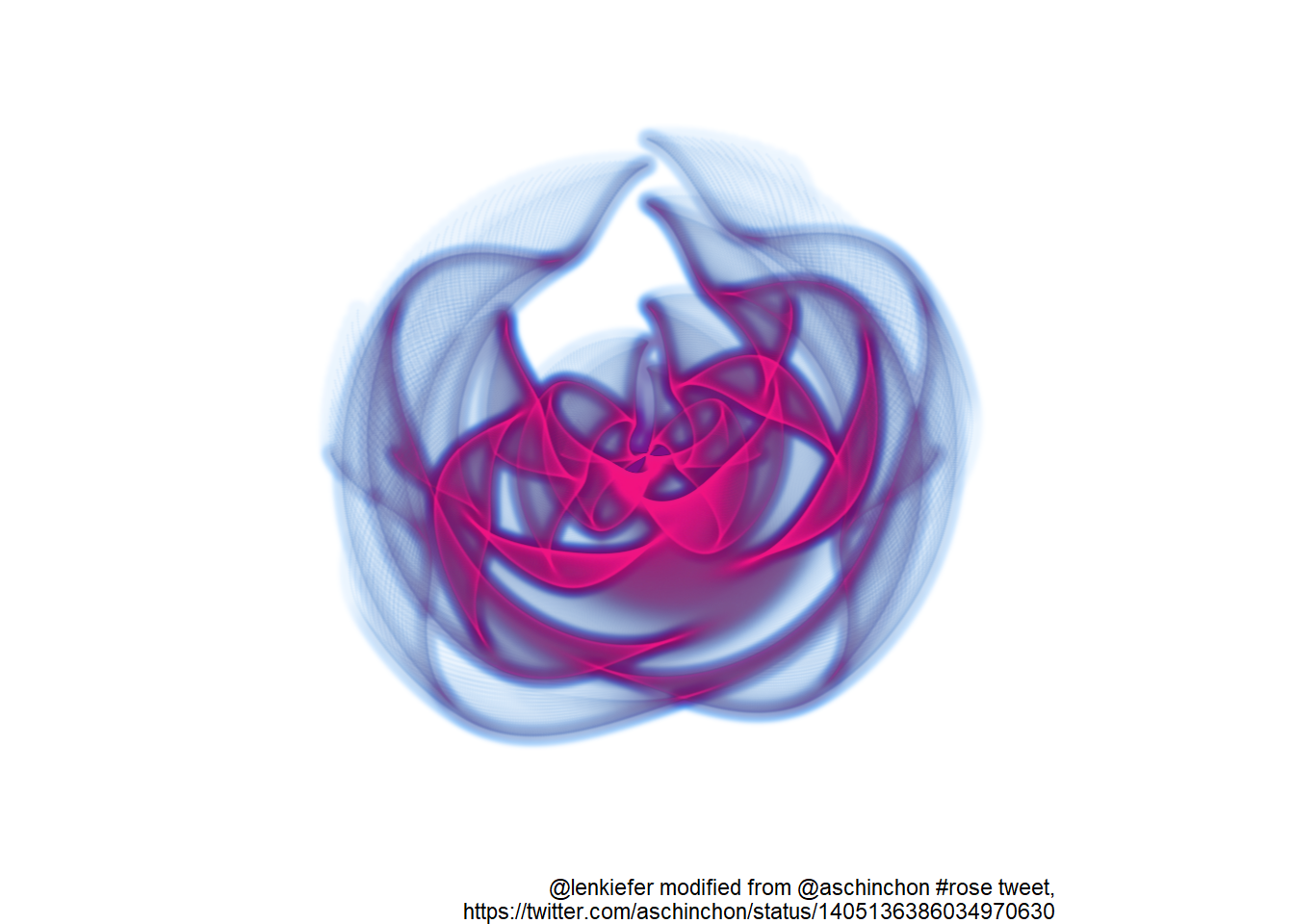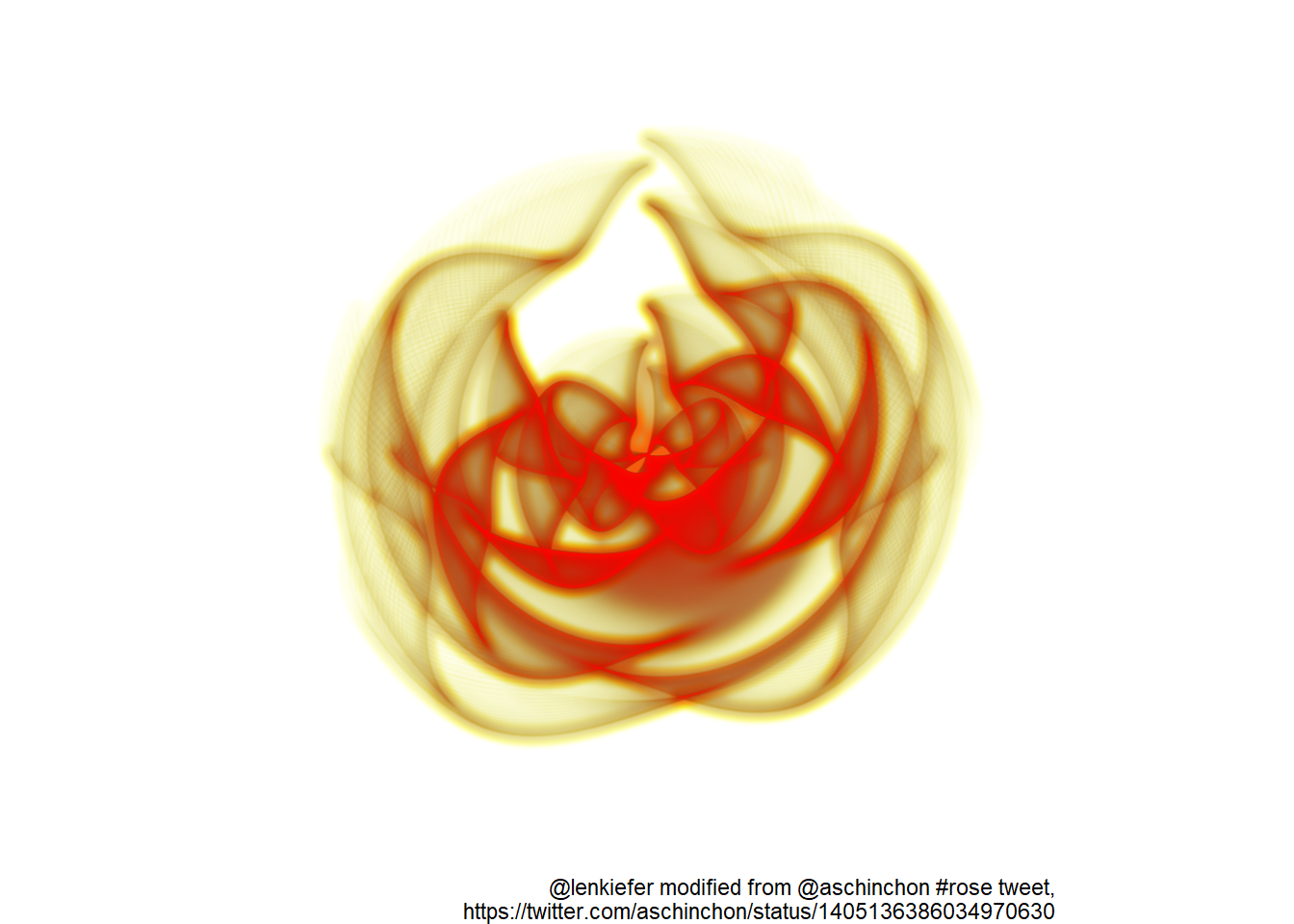# Rose chart in R

## A chart by any other name would still look as sweet

I want to share with you a fun rose chart. No not the Nightingale chart (see Jeff Shaffer). Rather we’re talking about a cool generative chart, that fit in a tweet from @aschinchon [https://twitter.com/aschinchon] :

I was inspired to use ggfx to add some features.

A little bit of setup:

library(tidyverse)
library(ggfx)

Original chart from the tweet

seq(-3,3,by=.01) %>%
expand.grid(x=., y=.) %>%
ggplot(aes(x=(1-x-sin(y^2)), y=(1+y-cos(x^2)))) +
geom_point(alpha=.05, shape=20, size=0)+
theme_void()+
coord_polar()I made my own tweet-sized remix

seq(-3, 3, by = .02) %>%
expand.grid(x = ., y = .) %>%
ggplot(aes(x = (1 - x - sin(y ^ 2)), y = (1 + y - cos(x ^ 2)))) +
ggfx::with_outer_glow(
geom_point(
alpha = .05,
shape = 20,
size = 0,
color = "white"
),
colour = "red",
expand = 10,
sigma = 3
) +
theme_void() +
coord_polar() +
labs(
caption = paste0(
"@lenkiefer modified from @aschinchon #rose tweet,",
)
)Then tried a few other permutations of colors and ggfx effects.

seq(-3, 3, by = .01) %>%
expand.grid(x = ., y = .) %>%
ggplot(aes(x = (1 - x - sin(y ^ 2)), y = (1 + y - cos(x ^ 2)))) +
ggfx::with_inner_glow(
colour = "dodgerblue",
expand = 5,
sigma =2,
ggfx::with_outer_glow(
geom_point(
alpha = .05,
shape = 20,
size = 0,
color = "deeppink"
),
colour = "purple",
expand = 10,
sigma = 2
)
) +
theme_void() +
coord_polar() +
labs(
caption = paste0(
"@lenkiefer modified from @aschinchon #rose tweet,",
),
title = ""
)seq(-3, 3, by = .01) %>%
expand.grid(x = ., y = .) %>%
ggplot(aes(x = (1 - x - sin(y ^ 2)), y = (1 + y - cos(x ^ 2)))) +
ggfx::with_inner_glow(
colour = "yellow",
expand = 5,
sigma = 2,
ggfx::with_outer_glow(
geom_point(
alpha = .05,
shape = 20,
size = 0,
color = "red"
),
colour = "#fe5305",
expand = 10,
sigma = 2
)
) +
theme_void() +
coord_polar() +
labs(
caption = paste0(
"@lenkiefer modified from @aschinchon #rose tweet,",
)